# Frequently Questioned Answers: Three Utilities

As a penultimate post in this series on problems that trigger a lot of doubt or opposition, we’ll look at one that is usually asked just as a “How do you do this?” question, but occasionally gets responses from people who argue for alternative interpretations. Our FAQ is at Three Houses, Three Utilities, which explains that the problem (in its pure form) is impossible to solve, giving two proofs of this fact. Unlike the angle trisection problem we looked at last time, there are not hordes of people claiming to have solved this one, but there are a few, and most of them simply misunderstand the point, taking it as a lateral thinking puzzle.

## An unsolvable problem

Getting All the Utilities to Each House

I've been stumped-here's the problem: Draw 3 one inch squares representing houses horizontally across the page.  Draw three circles, one under each square.  Number your squares and put a G for gas, E for electric, and W for water in each circle.  Your job is to connect each utility to each home WITHOUT crossing any lines. You may not pass a line through a house or other utility circle. Leave an inch and a half between each house. Put each circle an inch below each square.

Here is a picture (borrowed from the FAQ):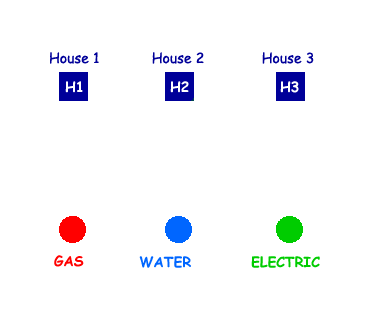So we have to draw nine (curved) lines, connecting each dot at the bottom to each of the houses. Here is an attempt: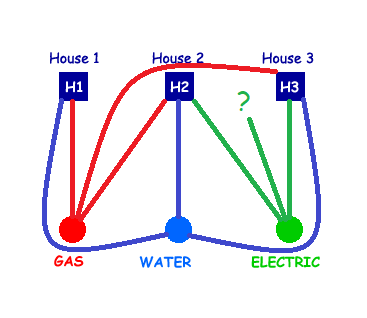We are missing one line, from Electric to House 1. The line we started is trapped between the green and red lines, one of which it would have to cross to get to the house.

It’s important to keep in mind that this is a pencil-and-paper puzzle, not a real-world problem; real electric lines can go in the air and pipes can be at different levels in the ground, so crossing is not a problem. Also, we are thinking of each square or dot as a single entity, effectively a point, so we can’t pass a pipe through a building as we might in real life; nor can we join wires into a loop from house to house. (All these are things people have written to us to suggest.) We are thinking of it as an abstract problem in graph theory, which deals with points joined by curves.

If you keep trying, you will keep failing the same way, until you should wonder, as Mr. Borstein probably did above, whether you are just missing something, or there is something wrong with the problem itself.

Doctor Ken replied briefly:

I hope I can make you feel a little better about the fact that you couldn't solve it, because there is no solution possible.

What's neat (and you might want to pass this along as a challenge to your students) is that while the problem can't be solved in the Euclidean plane, it CAN be done on the surface of a torus (a doughnut).  See if your students can figure out why.

This is the first level of answer: Don’t worry, it’s not just you!

When a mathematician finds that something feels impossible, he has two next thoughts: Can I prove it really is impossible? And, can I modify the conditions to make it possible? Those are the two points Doctor Ken made.

## Cutting a hole

A similar question came in in 1996:

Graph Without Crossing Lines

There are three houses           1        2           3
and
there are three utilities       gas     water      electric

How can you connect the three houses individually to the three utilities without crossing your lines?

(You have to draw a line from each house to each of the utilities without the lines ever crossing.)

Hi, sorry to tell you this, but that problem is impossible on most normal surfaces.  The only one I know of where it will work is what is called a torus--which is basically a doughnut-shaped figure with a hole in the center.

If you can, try the problem again on a surface like that and see what happens.  Since a torus might be hard to come by, you can cut a hole in the center of the paper and allow lines to go onto both sides and through the hole onto the other side.

Why do you think it works in this shape?

A piece of paper with a hole in it, when you have permission to go around to the other side both at the hole and at the edges of the paper, is equivalent to a torus. Here is an example solution, where we have fixed the problem in the attempt I showed above by taking that last line through the hole: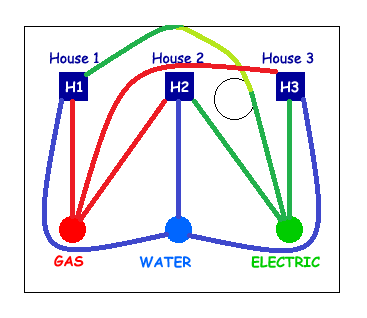(Here the light green represents a green line on the back of the paper.)

By the way, the hole is necessary, not just the permission to wrap around; without the hole, wrapping around just turns the paper into the equivalent of a sphere, which doesn’t help.

The FAQ shows such a solution on an actual torus. Now, let’s look at one of the proofs that it can’t be done on a plane.+

## A proof

Here is a question from 2002:

Water, Gas, Electricity to Three Homes Puzzle

This is a puzzle that I have tried over and over and over to try to get.  You have the three letters W G E  arranged in any position and you also have 3 circles (homes) arranged in any position.  You have to draw a line from each of the W G and E to each of the 3 circles without crossing lines.  You can put the letter and shapes in any shape or positions to do this.  I have always got to one line left over and over and over.  I believe that this is an impossible one to solve.  If you figure it out, can you tell me what order you put down the lines since that's half of how you do the problem.

I HATE THIS PUZZLE!

Doctor Anthony provided not only the answer, but a proof (similar to one of the two proofs in the FAQ):

We have 3 utilities A, B, C and 3 houses 1, 2, 3 as shown

A    B    C

1    2    3

and we must join each letter to each number without any of the lines crossing each other.

This problem comes under the heading of graph theory.

You have probably heard of Euler's formula for plane graphs.  If p = number of vertices, q = number of edges, r = number of regions then

p - q + r = 2

A plane graph has none of its edges intersecting except at a vertex.

For example, in a square, you have p = 4, q = 4, r = 2 (one region inside the square, one region outside the square) and

p - q + r = 4 - 4 + 2 = 2   as required.

The proof of Euler's formula is straightforward and can be found in any textbook on basic graph theory.  We now use it to show that it is impossible to join the three letters to the three numbers without any intersections of the edges.

We previously discussed Euler’s Formula. Commonly we use the letters v, e, and f for the number of vertices, edges, and faces (regions).

We prove the result by contradiction.  Suppose that it is possible to draw the figure without any intersections of the edges.

We sum the number of edges lying on the boundary of each region over all r regions and denote this number by N.  Since no edges join letters to each other or numbers to each other, the graph contains no triangles, so it follows that N >= 4r  [To illustrate this, think again of a square.  The inner region has 4 edges on its boundary.  The outer region has 4 edges on its boundary and so N = 8.  If we had a triangle then N would be 3+3 = 6 but as stated above there are NO TRIANGLES.]

Here is an example of a region with four edges: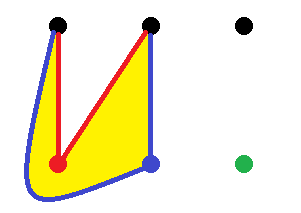On the other hand N counts each edge AT MOST twice (an edge can only have 1 or 2 regions on either side), so N <= 2q (= 2 x 9 = 18).

We had N >= 4r   so

4r <= N <= 18

r <= 9/2  (=4.5)   ......(1)

However by Euler's formula,

p - q + r = 2

6 - 9 + r = 2

r = 5  ..................(2)

Comparing (1) and (2) we see that there is a contradiction and our assumption that the figure is a plane graph is clearly impossible.

It follows that the letters and numbers cannot be joined without at least one intersection of two lines.

This also provides an explanation for the fact that the puzzle can be solved on a torus: the equivalent of Euler’s formula there is p – q + r = 0, from which we get r = 3, and there is no contradiction.

Asking whether the problem can be solved on other surfaces is interesting math. But many people claim to be able to solve the problem by some “trick”. Here is someone in 1999 who claimed to do it without “tricks”:

Three Houses, Three Utilities

I know that you have answered this before: the question about the three houses and the three utilities (gas, electricity, water).

Well, the guy who gave me this puzzle says there is a way of solving it in 2D, without any tricks. He says that it is simple, once you figure it out. I don't get it. Everywhere, it says that it can only be done using 3 dimensions. Can you solve it using 2 dimensions?  How?

Hmmm …

Doctor Rob answered with a clarification of the problem:

You can only solve this if you allow one of the utility lines to run through someone else's house, or through one of the other utility companies, which I suppose is possible, but is usually forbidden by the conditions of the puzzle.

Such evasions are generally considered tricks. Here is such a “solution”: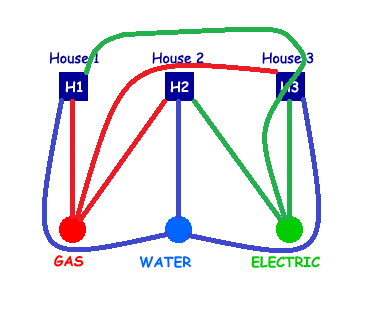I added a comment, listing the other basic tricks we’ve seen:

He may not call it a trick, but any solution that's really 2D (that is, done just by drawing non-intersecting curves on a flat sheet of paper) has to twist the rules somehow. He might, for example, draw the houses as rectangles and say that it's legal to open the front and back doors of one house and pass a pipe through. I call that a trick. Another trick is to solve it on the surface of a donut (a torus) and point out that any surface is itself 2-dimensional, even though it exists in a 3-dimensional space. Or you can allow going around to the other side of the paper through a hole, which is essentially the same thing.

But why are the rules what they are? How do we “know” that these tricks aren’t allowed? Because we know the genre of the problem, which is mathematical rather than a riddle. I continued:

When the problem is stated carefully in mathematical terms (continuous non-intersecting curves from each of three points to each of three other points), there's no solution; but presented in terms of houses and utilities (which are inherently three-dimensional), there are lots of ways to get around it.

So this problem, like some of the others (Boy or Girl? being the best example), is commonly stated as if it were a real-life problem to make it more relevant and impressive, but in doing so it can miss the point, running the risk of making math appear pedantic.

## Why not use trick answers?

Here is what another person (Bruce, unarchived) said in 2007 in arguing against our answer, claiming he had a solution:

How my solution works isn't based on mathematics or tricks - it simply takes advantage of the way the puzzle is stated (the rules). If there is no rule barring the action, then it is "legal" to do it. The reason nobody has "figured out" the puzzle is A) Not paying attention to the rules. B) People keep re-writing the puzzle and adding their own (new) rules to it. And C) People are missing the very simple solution because they are paying more attention to what IS being stated than what ISN'T being stated in the rules. For instance - Howard Dudeney did not say you could not "leave the plane" as you have asked me here. So while the puzzle as stated probably before you were born was solvable due to that opening - you have changed the rules in order to "make" it unsolvable. I have seen other variations and people trying to make it stricter (hence "unsolvable"), but even so I still have come up with several methods of solving the puzzle, because there's always a "loophole" in the rules. Let me know if you would like to see - I bet you'll be unhappy about it, because it isn't some "high math" solution... just plain observance.

Henry Dudeney was a popular puzzle creator in the early 20th century, who put this puzzle in its current form, in 1913. (For more information, see Wikipedia.) The notes there lead to this copy of the original puzzle, in Strand Magazine: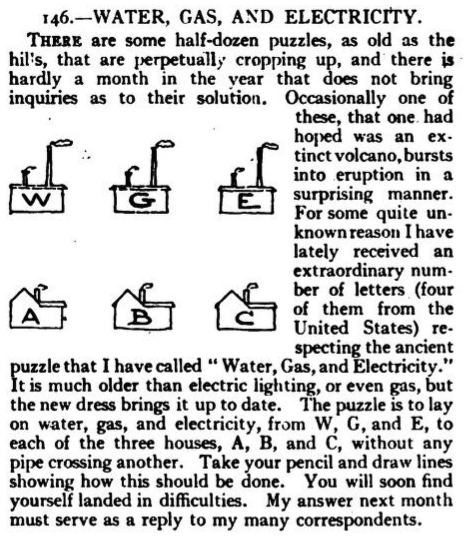He doesn’t present it in the abstract mathematical form; and in fact, his solution is the same trick answer we’ve seen: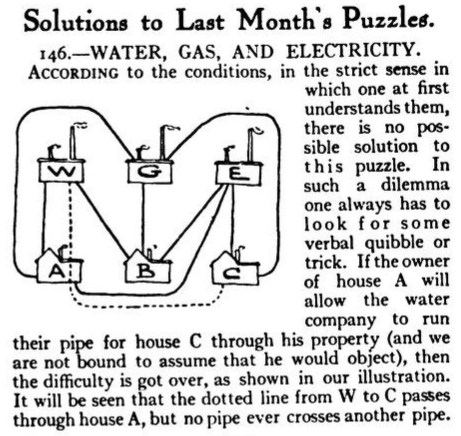Quite likely, this is also Bruce’s solution. How do we justify disallowing it, when Dudeney accepts it? Doctor Ian responded:

I think you might be missing the point of the problem.  What's interesting to mathematicians is the abstract problem, defined purely in terms of mathematical concepts, and there are no loopholes there. Of course, there's also very little there to interest anyone who isn't a mathematician.

It just gets expressed in terms of things like houses and utilities to make it more understandable to the general public.  Of course, that requires setting up analogies, and anytime you set up an analogy, it creates openings for pushing it so hard that it breaks.  That's inherent in the concept of an analogy, so it's not terribly interesting when it happens.

Now, if you'd like to forget about houses and utilities, and show that it's possible to construct a planar graph from each of three nodes to each of three other nodes, then that's interesting.  Can you do that?

That is, to a mathematician the puzzle is really an opportunity to prove something abstract about points and lines (that is, about graph theory); and this puzzle, in that form, predates Dudeney. Dudeney was interested in puzzles, so in his context the trick is what makes it fun. To each his own!

By the way, someone wrote this in 2005 (unarchived, and in fact unanswered):

Dudeney wrote the utilities problem long before running water, gas, and electricity were found in houses. What was his original problem back in 1917, since it is impossible to believe he wrote it the way it is found today?

The assumption is wrong, of course; but it would be interesting to see the older forms Dudeney referred to. Possibly they didn’t allow his trick.

## Why torment me with an unsolvable problem?

Some people write to us having seen the puzzle long ago without, apparently, being told the point (that it can be proved to be impossible) or allowed to use a trick. That can become unintentionally sadistic! Here is one, from Medora in 2003:

My question is mostly rhetorical.  When I was 12, this question was given to my math class by Mr. Ostrander with the words, "See if you can solve it."  He didn't know how stubborn I was.  I worked on this problem for years, until it dawned on me that I had been duped--the problem was insoluble in two dimensions.  (Note that I was always a "humanities" type student: literature, music, art, history, philosophy, and now theology are my areas.)  Thank you for providing an answer for me 43 years after I began struggling with this.  I came across your site by accident while looking for the secret of the universe.  I just wonder why math teachers feel the need to torment people like me for decades!

I know that your question is rhetorical; nonetheless, I would like to suggest an answer to you that may make you feel a bit more positively about Mr. Ostrander.

I believe, as perhaps Mr. Ostrander did, that oftentimes finding the answer to a problem is not as important as the process of seeking the answer.  This process requires tenacity (which apparently you have), creativity, the willingness to try new and different ideas, and oftentimes the process opens doors to new ways of thinking.

I suggest that your success in the areas you listed above may have been enhanced by the time you spent struggling with the "3 utilities to 3 houses" problem.

I hope this makes some sense to you.  At any rate, thank you for your letter and best wishes in your future studies.  Write back if you would like to discuss this some more.

But I have to say, it seems inappropriate not to tell students the point! We need an “out” – one of those “open doors”, which might be either a lateral-thinking trick, or a way to prove impossibility.

In closing, here is something I wrote in 2011:

Although this puzzle is stated as a real-world problem, its interest to mathematicians is in its abstract form: Given two sets of three points on a plane, it is impossible to make continuous non-intersecting curves joining each of the first three points to each of other three points. What makes this interesting is that we can prove that it is impossible -- not just give up after trying, and just believe that it is impossible.

I think this is the important point that many people miss, because they don't see the problem from a mathematician's perspective. Trick solutions are fun, but proofs of unexpected facts are amazing!

### 6 thoughts on “Frequently Questioned Answers: Three Utilities”

1.I solved it please get back to me

1.Most likely your “solution” falls into one of the categories I discussed. But feel free to show what you did, and maybe it will add something to the discussion.

2.Bunu nasil çozdunuz?

1.You asked in Turkish, “How did you solve this?”. We’ll see whether Carlos has more to say. But as I said, it was probably a “non-solution” like those I discussed.

2.Do the rules state that the three home must be in a row with each utility also in a row, but across from the homes?
For example:
H H H
w e g

Or can we place each home and each utility where we want on the paper? Because if we can place each home and each utility where we want on the paper, then this puzzle is solvable.
For example:
w H e
H g H

1.Actually, that doesn’t make any difference.

In its pure mathematical form, this is a problem of topology; that is, it is only about connections, not actual locations. If you had a real solution in your arrangement, you could drag the items around on the plane into the standard arrangement, and it would still be a solution. This is an inherent property of the plane. (To put it another way, the proof we gave does not depend on the locations of the houses and utilities (nodes in a planar graph.)

This site uses Akismet to reduce spam. Learn how your comment data is processed.